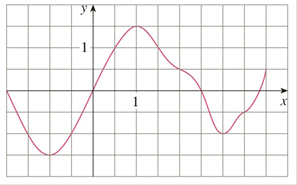Chapter 4.2, Problem 6E

Chapter
Section
Textbook Problem

# The graph of g is shown. Estimate ∫ − 2 4 g ( x ) d x with six subintervals using (a) right endpoints, (b) left endpoints, and (c) midpoints.To determine

Part (a):

To estimate:

-24gxdx with the six subintervals by using the right endpoints.

Explanation

1) Concept:

Use the theorem(4) to estimate the given integration by using the right endpoints.

2) Theorem (4):

If f is an integrable function on [a, b] then

abf(x)dx=limni=1nf(xi)x

where  x=b - an,n is the number of the subintervals and xi=a+ix

and i=1nf(xi*)x is the Riemann sum formula.

3) Given:

The graph:

4) Calculation:

Here, n=6, a=-2 and b=4.

So, the width of the subintervals is

x=b-an

x=4-(-2)6

x=66=1

Use the width x=1 to form the subintervals.

-2, -1,-1, 0,0, 1, 1, 2, 2, 3 and 3, 4

The right endpoints are x1=-1, x2=0, x3=1, x4=2, x5=3, x6=4

since the we are using right end point xi*=

To determine

Part (b):

To estimate:

-24gxdx with the six subintervals by using the left endpoints.

To determine

Part (c):

To estimate:

-24gxdx with the six subintervals by using the midpoints

### Still sussing out bartleby?

Check out a sample textbook solution.

See a sample solution

#### The Solution to Your Study Problems

Bartleby provides explanations to thousands of textbook problems written by our experts, many with advanced degrees!

Get Started

#### In Exercises 4562, find the values of x that satisfy the inequality (inequalities). 49. 6 x 2 4

Applied Calculus for the Managerial, Life, and Social Sciences: A Brief Approach

#### In Problems 7-34, perform the indicated operations and simplify. 15.

Mathematical Applications for the Management, Life, and Social Sciences

#### Subtract: 4000484

Elementary Technical Mathematics

#### In Exercises 14, find the slope of the line shown in each figure.

Finite Mathematics for the Managerial, Life, and Social Sciences

#### For

Study Guide for Stewart's Multivariable Calculus, 8th